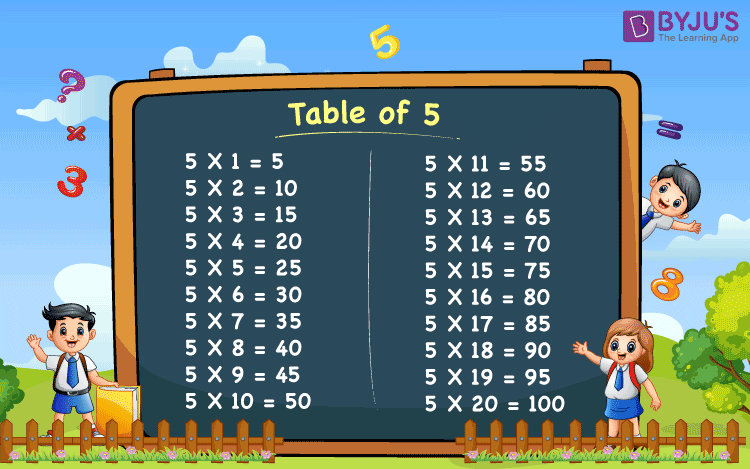# Table of 5

Table of 5 is provided below that is essential for the students to memorize the table. The multiples of 5 up to 20 helps students in fast multiplication as well as in the time-based competitive examination to attempt more question. It is suggested to everyone that they learn the multiplication table given below.

## Table of 5## Multiplication Table of 5

 5 x 1 = 5 5 x 2 = 10 5 x 3 = 15 5 x 4 = 20 5 x 5 = 25 5 x 6 = 30 5 x 7 = 35 5 x 8 = 40 5 x 9 = 45 5 x 10 = 50 5 x 11 = 55 5 x 12 = 60 5 x 13 = 65 5 x 14 = 70 5 x 15 = 75 5 x 16 = 80 5 x 17 = 85 5 x 18 = 90 5 x 19 = 95 5 x 20 = 100

Get More Maths Tables:-

Test your knowledge on Table Of 5